# 1661 A Little About Palindromes

### Today’s Puzzle:

A number is a palindrome if it reads the same backward as it does forward. For example, 1661 is a 4-digit palindrome. Today’s puzzle asks you to explore number palindromes and their factors.Half of the time when a 4-digit palindrome is divided by 11, we get a 3-digit palindrome. Why does that happen?Are there 3-digit palindromes that were NOT included in the Divided by Eleven column in the table above?

All 2-digit palindromes are divisible by 11. They are 11, 22, 33, 44, 55, 66, 77, 88, 99.

There are only eight 3-digit palindromes that are divisible by 11. They are 121, 242, 363, 484, 616, 737, 858, 979. Some 3-digit palindromes are prime numbers. Others are divisible by 101 or 111. Still, there are plenty of 3-digit palindromes that are composite numbers but not divisible by 11, 101, or 111.

Most 5-digit palindromes are NOT divisible by 11. I was able to construct one that is, 76967, because the red digits minus the blue digits are 2312 = 11, a number divisible by 11.

Here’s another: 81818. It works because 242 = 22, a number divisible by 11.

What 5-digit palindrome can you construct that is divisible by 11?

Will an N-digit palindrome be divisible by 11? What difference does it make if N is an even number or if N is an odd number?

### Factors of 1661:

1661 is divisible by 11 because the red digits minus the blue digits equal 0, a number divisible by 11.

• 1661 is a composite number.
• Prime factorization: 1661 = 11 × 151.
• 1661 has no exponents greater than 1 in its prime factorization, so √1661 cannot be simplified.
• The exponents in the prime factorization are 1 and 1. Adding one to each exponent and multiplying we get (1 + 1)(1 + 1) = 2 × 2 = 4. Therefore 1661 has exactly 4 factors.
• The factors of 1661 are outlined with their factor pair partners in the graphic below.### More about the Number 1661:

1661 is also a palindrome in base 18:
1661₁₀ = 525₁₈ because
5(18²) + 2(18¹) + 5(18º) =
5(324) + 2(18) + 5(1) =
1620 + 36 + 5 =
1661.

# 1462 Has a Palindromic Factor Pair

There are only forty-five numbers between 100 and 10,000 that have palindromic factor pairs. That’s really not very many.

1462 is one of those numbers. That is pretty amazing, all by itself, but 1462 is also only four numbers more than another number on the list. That’s even more amazing!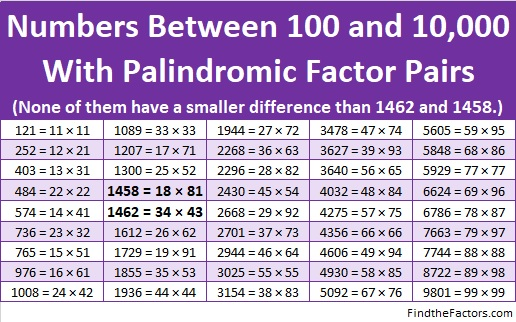Here are some more facts about the number 1462:

• 1462 is a composite number.
• Prime factorization: 1462 = 2 × 17 × 43
• 1462 has no exponents greater than 1 in its prime factorization, so √1462 cannot be simplified.
• The exponents in the prime factorization are 1, 1, and 1. Adding one to each exponent and multiplying we get (1 + 1)(1 + 1)(1 + 1) = 2 × 2 × 2 = 8. Therefore 1462 has exactly 8 factors.
• The factors of 1462 are outlined with their factor pair partners in the graphic below.1462 is the hypotenuse of one Pythagorean triple:
688-1290-1462 which is (8-15-17) times 86

# 898 and Level 2

27² + 13² = 898. That means that 898 is the hypotenuse of a Pythagorean triple:

• 560-702-898, which is 2 times (280-351-449).

898 reads the same way frontwards and backwards so it is a palindrome in base 10.

It is also palindrome 747 in BASE 11 because 7(11²) + 4(11) + 7(1) = 898

AND it is palindrome 1G1 in BASE 23 (G is 16 in base 10) because 1(23²) + 16(23) +1(1) =898.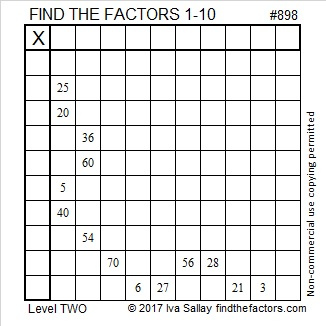Print the puzzles or type the solution on this excel file: 10-factors-897-904

• 898 is a composite number.
• Prime factorization: 898 = 2 × 449
• The exponents in the prime factorization are 1 and 1. Adding one to each and multiplying we get (1 + 1)(1 + 1) = 2 × 2 = 4. Therefore 898 has exactly 4 factors.
• Factors of 898: 1, 2, 449, 898
• Factor pairs: 898 = 1 × 898 or 2 × 449
• 898 has no square factors that allow its square root to be simplified. √898 ≈ 29.9666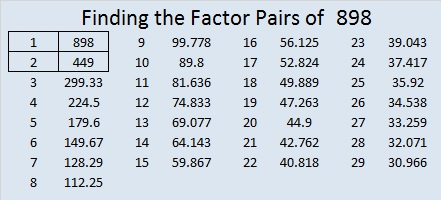# Palindrome 868 Has a Fun Square Root

Obviously, 868 is a palindrome in base 10. It has some interesting representations in some other bases, too:

• 868 is 4004 in BASE 6, because 4(6³) + 0(6²) + 0(6¹) + 4(6º) = 868
• SS in BASE 30 (S is 28 base 10), because 28(30) + 28(1) = 28(31) = 868
• S0 in BASE 31, because 28(31) = 868

From OEIS.org I learned that all the digits from 1 to 9 make up the first nine decimal places of √868, so I decided to make a gif showing that fun fact:make science GIFs like this at MakeaGif
• 868 is a composite number.
• Prime factorization: 868 = 2 × 2 × 7 × 31, which can be written 868 = 2² × 7 × 31
• The exponents in the prime factorization are 2, 1, and 1. Adding one to each and multiplying we get (2 + 1)(1 + 1)(1 + 1) = 3 × 2 × 2 = 12. Therefore 868 has exactly 12 factors.
• Factors of 868: 1, 2, 4, 7, 14, 28, 31, 62, 124, 217, 434, 868
• Factor pairs: 868 = 1 × 868, 2 × 434, 4 × 217, 7 × 124, 14 × 62, or 28 × 31,
• Taking the factor pair with the largest square number factor, we get √868 = (√4)(√217) = 2√217 ≈ 29.461839725# 858 and Level 4

There are sixteen numbers less than 1000 that have four different prime factors. 858 is one of them, and it is the ONLY one that is also a palindrome. Thank you, OEIS.org for alerting us to that fact. No smaller palindrome has four different prime factors!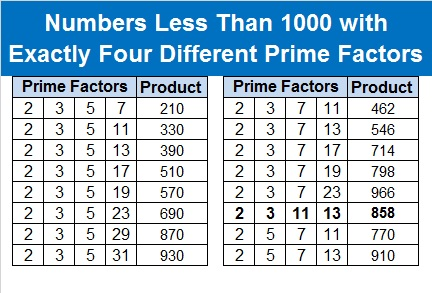The sixteen products on that chart each have exactly sixteen factors!

Here’s a Find the Factors 1-10 puzzle for you to solve: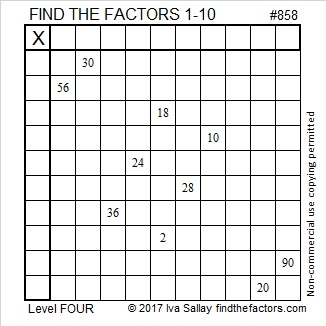Print the puzzles or type the solution on this excel file: 10-factors-853-863

Here’s a little more about the number 858:

858 is the hypotenuse of a Pythagorean triple: 330-792-858

• 858 is a composite number.
• Prime factorization: 858 = 2 × 3 × 11 × 13
• The exponents in the prime factorization are 1, 1, 1, and 1. Adding one to each and multiplying we get (1 + 1)(1 + 1)(1 + 1)(1 + 1) = 2 × 2 × 2 × 2 = 16. Therefore 858 has exactly 16 factors.
• Factors of 858: 1, 2, 3, 6, 11, 13, 22, 26, 33, 39, 66, 78, 143, 286, 429, 858
• Factor pairs: 858 = 1 × 858, 2 × 429, 3 × 286, 6 × 143, 11 × 78, 13 × 66, 22 × 39, or 26 × 33
• 858 has no square factors that allow its square root to be simplified. √858 ≈ 29.291637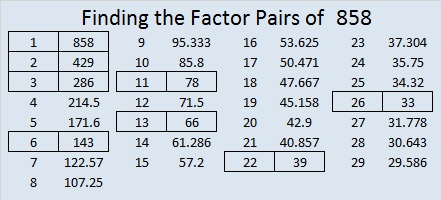# 797 and Mathematical Ways to Love

Mathwithbaddrawings.com has some thoughtful and entertaining Ways to Tell a Mathematician that you love them.

Artful Maths wrote a post that includes beautiful mathematical origami valentines and a “string art” cardioid that is made with a pencil instead of string.

This puzzle could be another mathematical way to show some love: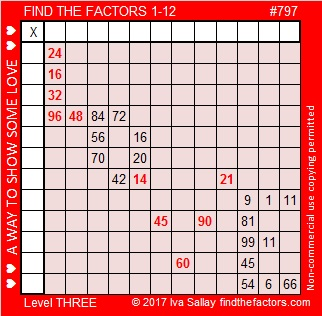Print the puzzles or type the solution on this excel file: 12-factors-795-799

Here are a few facts about the number 797:

797 is a palindrome in three bases:

• 797 BASE 10 because 7(100) + 9(10) + 7(1) = 797
• 565 BASE 12 because 5(144) + 6(12) + 5(1) = 797
• 494 BASE 13 because 4(169) + 9(13) + 4(1) = 797

But there’s one more palindromic fact about the number 797: It is the sum of two square numbers that are also palindromes!

• 797 = 121 + 676. Note that 11² = 121 and 26² = 676.

Since it is the sum of two squares, 797 will also be the hypotenuse a Pythagorean triple:

• 555 – 572 – 797 calculated from 26² – 11², 2(26)(11), 26² + 11².

797 is the sum of three squares seven different ways:

• 28² + 3² + 2² = 797
• 27² + 8² + 2² = 797
• 24² + 14² + 5² = 797
• 24² + 11² + 10² = 797
• 22² + 13² + 12² = 797
• 21² + 16² + 10² = 797
• 20² + 19² + 6² = 797

797 is also the sum of the 15 prime numbers from 23 to 83:

• 23 + 29 + 31 + 37+ 41 + 43 + 47 + 53 + 59 + 61 + 67 + 71 + 73 + 79 + 83 = 797

Here is the most basic information about the number 797:

• 797 is a prime number.
• Prime factorization: 797 is prime.
• The exponent of prime number 797 is 1. Adding 1 to that exponent we get (1 + 1) = 2. Therefore 797 has exactly 2 factors.
• Factors of 797: 1, 797
• Factor pairs: 797 = 1 x 797
• 797 has no square factors that allow its square root to be simplified. √797 ≈ 28.231188.

How do we know that 797 is a prime number? If 797 were not a prime number, then it would be divisible by at least one prime number less than or equal to √797 ≈ 28.2. Since 797 cannot be divided evenly by 2, 3, 5, 7, 11, 13, 17, 19, or 23, we know that 797 is a prime number.

Here’s another way we know that 797 is a prime number: Since  its last two digits divided by 4 leave a remainder of 1, and 26² + 11² = 797 with 26 and 11 having no common prime factors, 797 will be prime unless it is divisible by a prime number Pythagorean triple hypotenuse less than or equal to √797 ≈ 28.2. Since 797 is not divisible by 5, 13, or 17, we know that 797 is a prime number.# 727 Enjoy Some Sparkling Cider!

Have a happy New Year’s Eve! My husband and I will be enjoying some Sparkling Apple-Pear. I invite you to have some, too.Perhaps the goblet in the puzzle can start off your festivities.Print the puzzles or type the solution on this excel file: 12 Factors 2015-12-28

—————————————————————————————————

• 727 is a prime number.
• Prime factorization: 727 is prime.
• The exponent of prime number 727 is 1. Adding 1 to that exponent we get (1 + 1) = 2. Therefore 727 has exactly 2 factors.
• Factors of 727: 1, 727
• Factor pairs: 727 = 1 x 727
• 727 has no square factors that allow its square root to be simplified. √727 ≈ 26.9629375.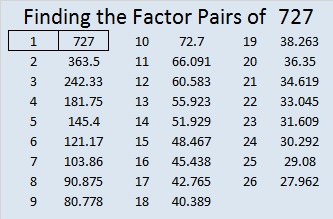727 is a palindrome in base 10 and one other base:

• 727 BASE 10; note that 7(100) + 2(10) + 7(1) = 727.
• 1B1 BASE 22 (B = 11 base 10); note that 1(22²) + 11(22) + 1(1) = 727.

OEIS.org informs us that 727² = 528529, a number whose digits can be split in half to make two consecutive numbers.

Since 727 is a prime number, there is only one way it can be expressed as the sum of consecutive numbers: 363 + 364 = 727.

—————————————————————————————————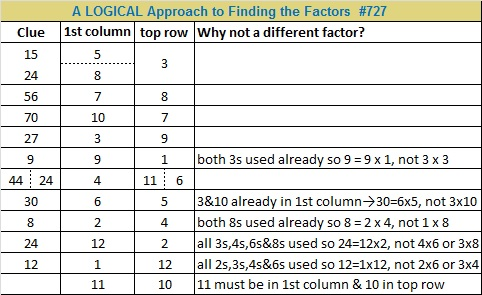# When is 690 a Palindrome? In Base 16 and Base 29.

• 690 is a composite number.
• Prime factorization: 690 = 2 x 3 x 5 x 23
• The exponents in the prime factorization are 1, 1, 1, and 1. Adding one to each and multiplying we get (1 + 1)(1 + 1)(1 + 1)(1 + 1) = 2 x 2 x 2 x 2 = 16. Therefore 690 has exactly 16 factors.
• Factors of 690: 1, 2, 3, 5, 6, 10, 15, 23, 30, 46, 69, 115, 138, 230, 345, 690
• Factor pairs: 690 = 1 x 690, 2 x 345, 3 x 230, 5 x 138, 6 x 115, 10 x 69, 15 x 46, or 23 x 30
• 690 has no square factors that allow its square root to be simplified. √690 ≈ 26.267851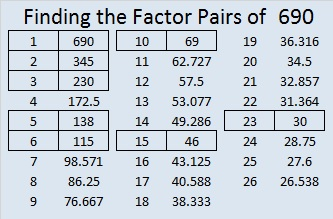Here is today’s factoring puzzle: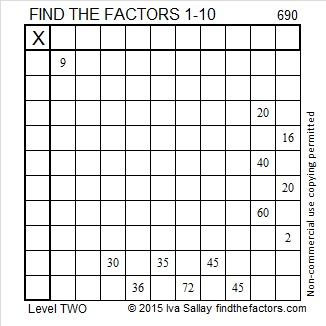Print the puzzles or type the solution on this excel file: 10 Factors 2015-11-23

———————————————————————————

Here is a little more about the number 690:

690 is the sum of the six prime numbers from 103 to 131. Do you know what all of those prime numbers are?

690 is also the hypotenuse of Pythagorean triple 414-552-690. What is the greatest common factor of those three numbers?

In BASE 10 we use the digits 0, 1, 2, 3, 4, 5, 6, 7, 8, and 9. For bases smaller than 10 we use only the digits less than that base. For bases greater than 10, we might use A to represent 10, B to represent 11, and so forth all the way to Z representing 35 in BASE 36.

690 is a palindrome in two bases that require us to use letters of the alphabet to represent it:

• 2B2 in BASE 16; note that 2(256) + 11(16) + 2(1) = 690
• NN in BASE 29; note that 23(29) + 23(1) = 690. (N is the 14th letter of the alphabet and 14 + 9 = 23)

NN looks like it is divisible by 11, but remember that 11 base 29 is the same as 30 in base 10.

———————————————————————————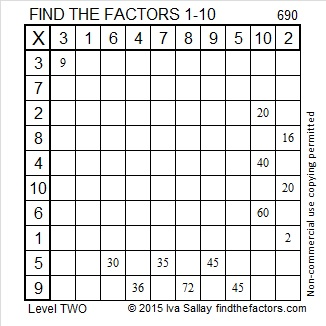# 686 and Level 5

686 is divisible by 2, not by 3, and not by 5. Is it divisible by 7? Let’s try a divisibility rule for 7’s: 68 – 2(6) = 56, a multiple of 7. Yes, 686 is divisible by 7.

686 is a palindrome in two bases:

• 686 in base 10; note that 6(100) + 8(10) + 6(1) = 681
• 222 in base 18; note that 2(324) + 2(18) + 2(1) = 681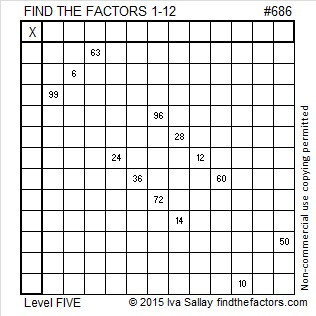Print the puzzles or type the solution on this excel file: 12 Factors 2015-11-16

—————————————————————————————————

• 686 is a composite number.
• Prime factorization: 686 = 2 x 7 x 7 x 7, which can be written 686 = 2 x (7^3)
• The exponents in the prime factorization are 1 and 3. Adding one to each and multiplying we get (1 + 1)(3 + 1) = 2 x 4 = 8. Therefore 686 has exactly 8 factors.
• Factors of 686: 1, 2, 7, 14, 49, 98, 343, 686
• Factor pairs: 686 = 1 x 686, 2 x 343, 7 x 98, or 14 x 49
• Taking the factor pair with the largest square number factor, we get √686 = (√49)(√14) = 7√14 ≈ 26.1916017.Here is a little cake to simplify √686: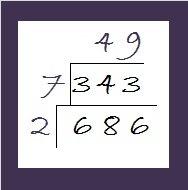Just take the square root of everything on the outside of the cake and multiply them together to get √686 = (√2)(√7)(√49) = 7√14

—————————————————————————————————# 626 Semordnilaps, Palindromes and Level 4

626 is the hypotenuse of the Pythagorean triple 50-624-626. What is the greatest common factor of those three numbers?

Today my son posted on facebook, “A word that when spelled backwards spells a different word is called a Semordnilap.” That’s a word I hadn’t heard before, and it also applies to phrases and sentences that form different phrases or sentences when read backwards.

“Semordnilap” is a semordnilap for the word “palindromes” which are words, phrases, sentences, and numbers that read the same forward and backward.

Palindrome sentences are sometimes made with words that are semordnilaps: was, saw, live, evil, on, no, desserts, stressed, stop, pots, tops, spot, diaper, repaid.

626 is a number that is a palindrome in several different bases:

• 10001 in base 5; note that (5^4) + 1 = 626.
• 626 in base 10
• 272 in base 16; note that 2(16^2) + 7(16) + 2 = 626
• 1DI in base 19, if “1” and “I” look the same, and too often they do. Note that 1(19^2) + 13(19) + 18 = 626
• 101 in base 25; note that (25^2) + 1 = 626
• 11 in base 625; note that 626 + 1 = 626

I guess we could say that in all other bases 626 is a semordnilap.

All of 626’s factors are palindromes, too.Print the puzzles or type the solution on this excel file: 12 Factors 2015-09-21

—————————————————————————————————

• 626 is a composite number.
• Prime factorization: 626 = 2 x 313
• The exponents in the prime factorization are 1 and 1. Adding one to each and multiplying we get (1 + 1)(1 + 1) = 2 x 2 = 4. Therefore 626 has exactly 4 factors.
• Factors of 626: 1, 2, 313, 626
• Factor pairs: 626 = 1 x 626 or 2 x 313
• 626 has no square factors that allow its square root to be simplified. √626 ≈ 25.019992.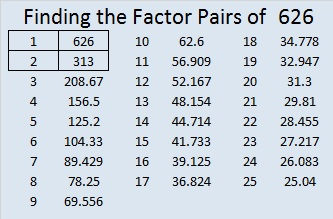—————————————————————————————————